# Constructing Understanding in Mathematics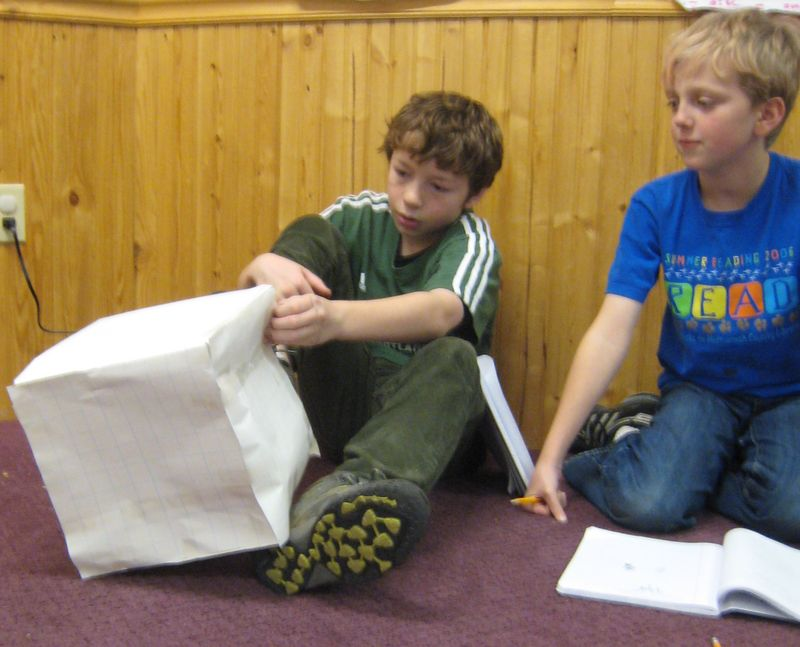The students in Levia’s math group have been working for the past couple months on developing an understanding of the associative property of mathematics by working with three-dimensional arrays.  The culminating investigation in their study was to determine the volume of 4 foot by 6 foot by 4 foot crate using two different non-standard units of measurement:  a 2 inch by 2 inch by 2 inch cube and a 4 inch by 4 inch by 4 inch cube.

How many 2 inch by 2 inch by 2 inch cubes will fit into a 4 foot by 6 foot by 4 foot crate?

How many 4 inch by 4 inch by 4 inch cubes will fit into a 4 foot by 6 foot by 4 foot crate?

For many of the students, the leap to a crate so large that it cannot be built out of paper or linker cubes was very hard to conceptualize.

In a classroom where children construct their own knowledge, the teacher has to be aware of times when knowledge is being constructed by her students and when it is not.  When it is not, it can be because the learner does not have or has not activated the schema he or she needs to connect to the new idea so that it makes sense.  Without that connection, the new idea cannot be integrated into the schema of that individual.  The idea cannot be constructed.

So, while we were in the middle of the crate investigation, we decided to tackle a similar problem, one we could touch and measure:  the stage in our classroom.

How can we use both standard and non-standard cubic units of measurement to calculate the volume of the stage?

The trick was, each pair of students had to use a different standard or non-standard cubic unit of measurement to do their calculation.  Here is what they chose:

• A cubic meter
• A cubic “Carlos” (a child in our school who is exactly 4 feet tall)
• A cubic foot
• A cubic “Marshall” foot (the length of a shoe-clad foot of a boy in our class, exactly 10 inches long)
• A cubic whiteboard (28 cubic inches)
• A cubic linker cube (2 cubic centimeters)
• A cubic yard
• A cubic math notebook

The idea behind doing this hands-on calculation of a space that we could see and touch was to start to be able to visualize what it looks like to fill up a large three dimensional, rectangular space with smaller and larger three dimensional, rectangular units.  Things we can see and touch are materials we can act on to activate relevant schema and to construct understanding.

First the children wondered:  what do these cubic units of measurement look like?  What is a cubic foot or a cubic Marshall foot for that matter?

“I need to build it [the Marshall cubic foot].  I am a visual learner.”  -MC

So he did.  Marshall’s foot is 10 inches long.

This 10 inch by 10 inch by 10 inch box is made out of square inch graph paper.  (Does it look bigger than you expected?  There are 1000 cubic inches in there!)

Here the class is trying to visualize a cubic meter by seeing how many mathematicians will fit inside.“Get a little taller.  I can’t fit another student on your heads.”  -MG

Just by looking at the cubic unit of measurement, can we guess how many of those it will take to cover the bottom layer of the stage?  Then how many will stack up to fill to the ceiling?

“If you take the box and dip it in ink and then stamp it on some paper, that is the [two dimensional] array of the bottom layer.”  -NF

The measuring of the stage took many forms.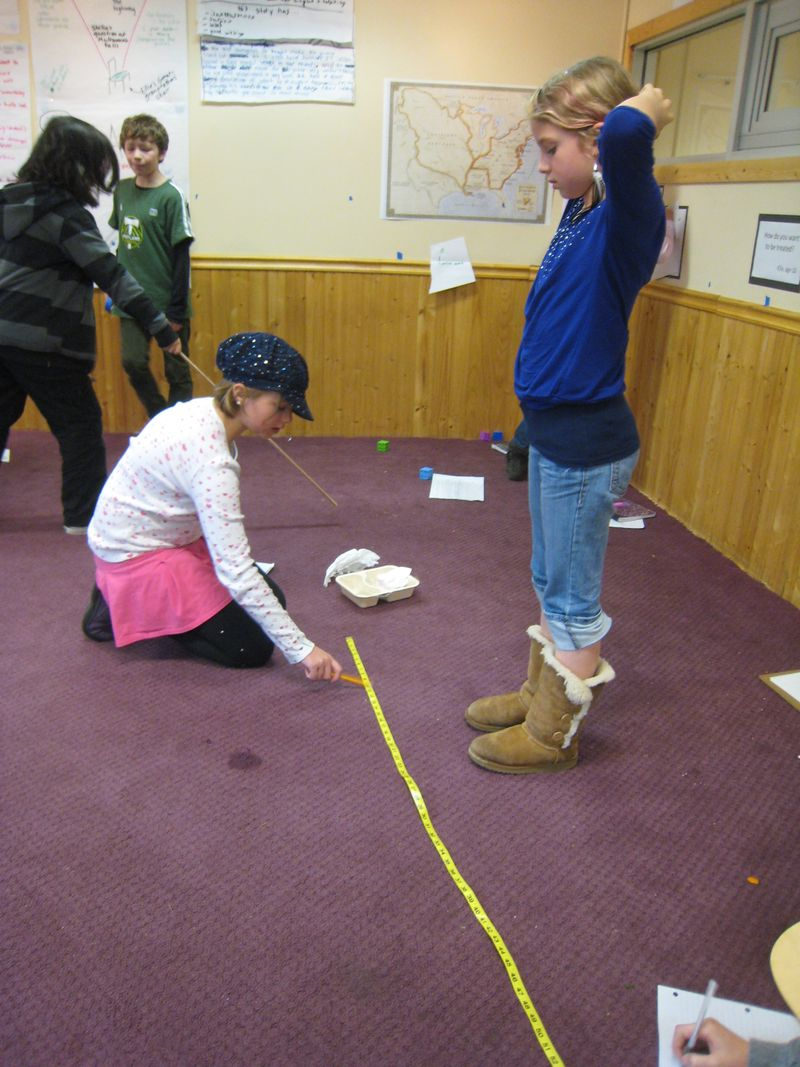“A linker cube is 2 cubic centimeters, so we just measured in centimeters and divided by two.”  -SBM

“We converted feet into the number of Carloses.”  -KB

And some used their own measuring tools.

This is a square foot: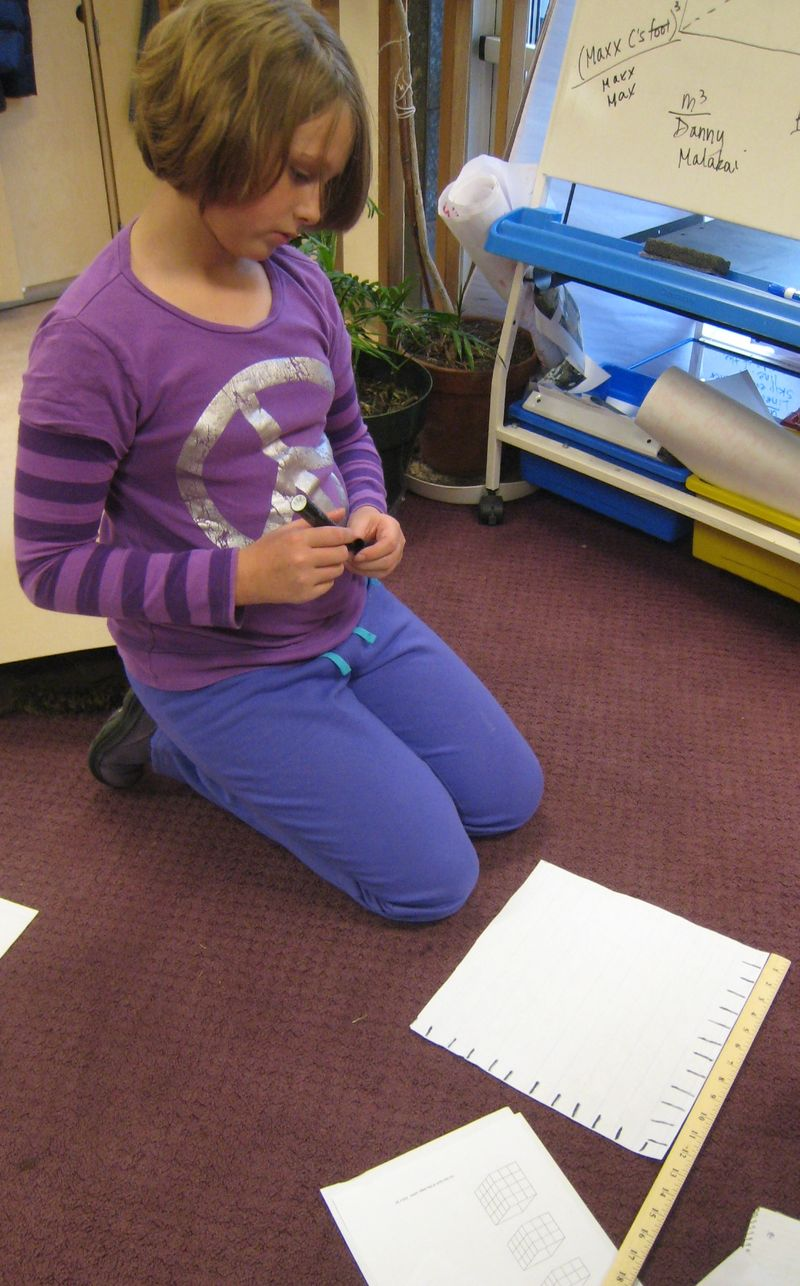This is a special ruler made by connecting together 10” segments of paper (Marshall feet):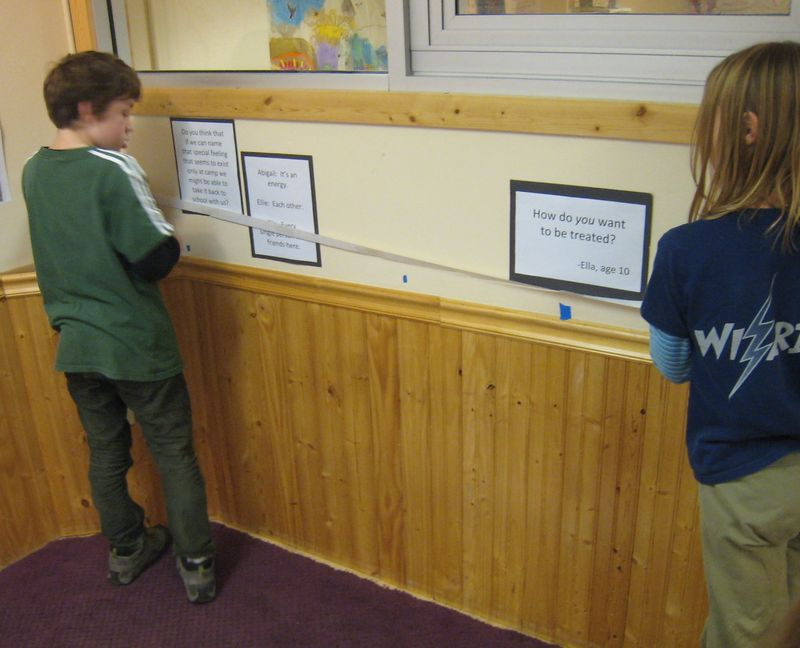“I am marking off every time there is another 28 inches (length of the whiteboard) with the blue tape.”  -EY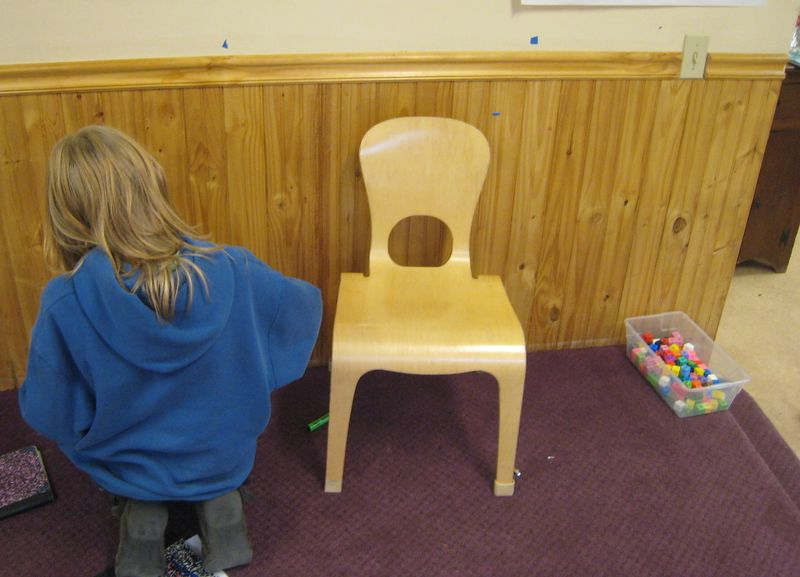Every student used the unit they were measuring do to their calculations.

We discovered equivalencies:  1 Carlos = 4 feet = 4 x 12 inches = 48 inches

And made their understanding of how those units filled the space of our stage clear.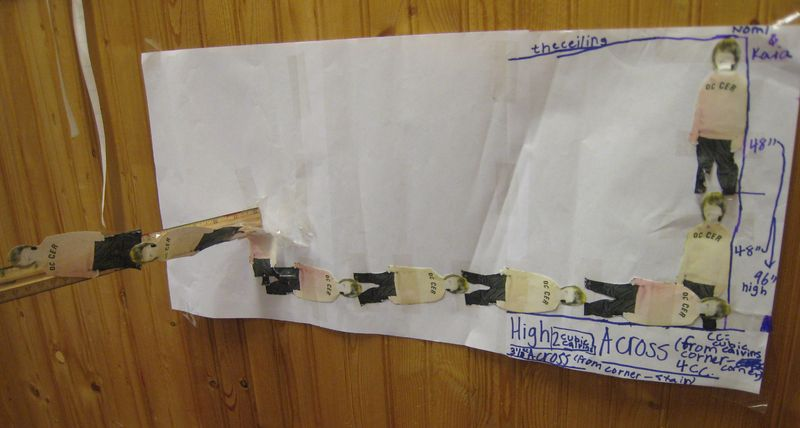Then the students recorded their thinking on mini-posters that they shared with each other.  As each mathematician shared, they walked the rest of the class through their thought process by sharing and discussing the following questions:

• What was your cubic unit of measurement?
• Can you give us an idea of what that looks like?
• Can we guess how many of those it will take to cover the bottom layer of the stage?
• Can you calculate how many of those it will take to cover the bottom layer of the stage?
• Can we guess how many layers of those cubic units we will need to get up to the ceiling?
• How can you calculate the actual number?
• Take a minute to visualize the space filled with those cubic units.  Can you imagine what they would look like?

After this, the students came back to the Crate Problem they had struggled with before.  Now, their schema required to connect to this new, abstract problem existed, and they could quickly and easily visualize how those cubes would fit into that crate and think of the problem in terms of equivalencies.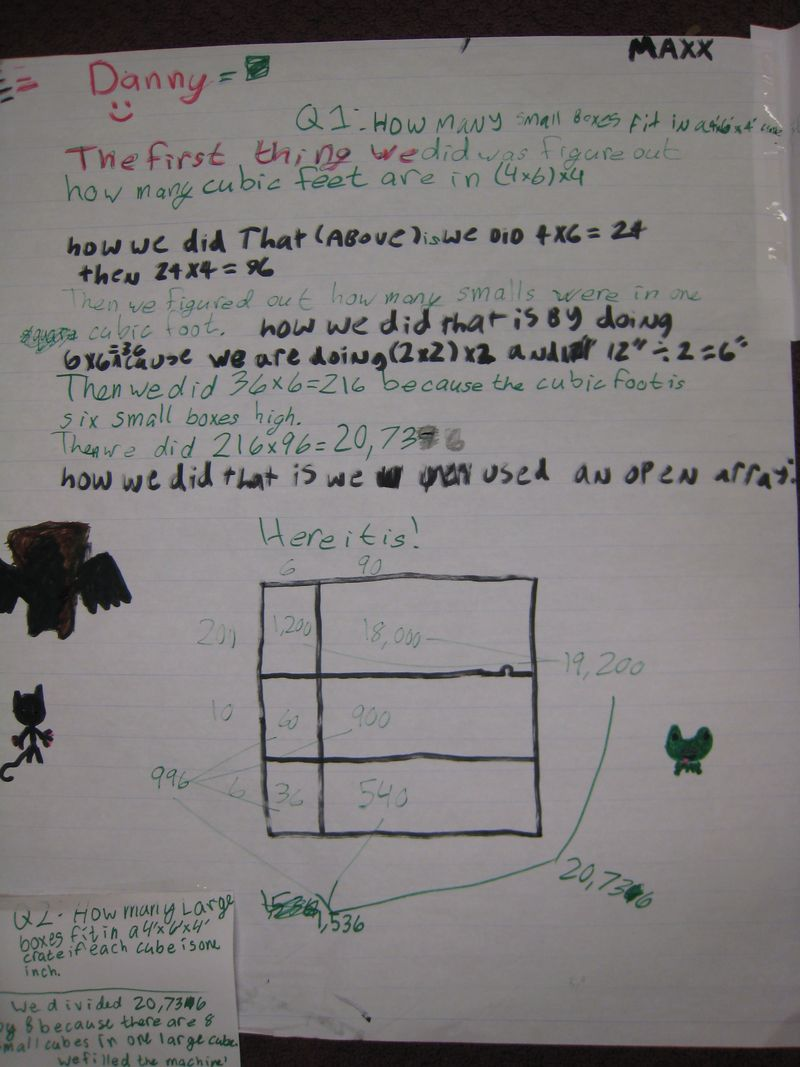What a great reminder this has been for me and for my students that:

[To construct ideas,] the effort that must be supplied is active and reflective thought.  If minds are not actively thinking, nothing happens.  If a potentially relevant idea that would add better meaning to a new idea is either not present in the learner’s mind or is not actively engaged, then that potential connection to the new idea simply will not be made.
(John A. Van de Walle, 2007)

How do we make sure relevant and supportive schema are present and are activated in our minds and in the minds of our students?  And, when they are not, what tools do we have to open and create pathways for connections?

December 06, 2011